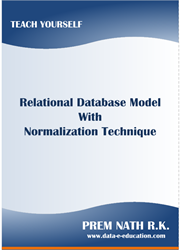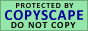FD & MVD Axioms Rules

MVD0 Complementation Rule

Let X, Y, and Z be sets of attributes that union is È and Y Ç Z Í X. Then X ®® Y if and only if X ®® Z.

MVD1 - Reflexivity Rule

If Y Í X, then X ®® Y

MVD2 - Augmentation Rule

If Z Í W and X ®® Y, then XW ®® YZ

MVD3 - Transitivity Rule

If X ®® Y and Y ®® Z, then X ®® Z - Y

The following rules follow from the above MVD rules.

MVD4 - Pseudotransitivity Rule

If X ®® Y and YW ®® Z, then XW ®® Z - YW

MVD5 - Union Rule

If X ®® Y and X ®® Z, then X ®® YZ

MVD6 - Decomposition Rule

If X ®® Y and X ®® Z, then X ®® Y Ç Z, X ®® Y - Z, and X ®® Z - Y

The rules of transitivity, pseudotransitivity, and decomposition are more restricted than the corresponding Functional Dependency rules.

FD-MVD1

If X ® Y, then X ®® Y

FD-MVD2

If X ®® Z and Y ® Z', where Z' Í Z and Y and Z are disjoint, then X ® Z'

FD-MVD3

If X ®® Y and XY ® Z, then X ® Z - YYour Comment To AUTHORDownload e-Book